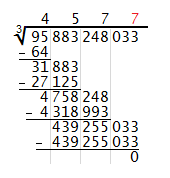# How to Compute Cube Roots by Hand

Prior to the widespread availability of hand calculators, most people calculated roots by looking up the values in a table or computing them by hand in a process similar to long division. The technique of calculating cube roots by hand is similar to the process of figuring square roots by hand, only the arithmetic is more difficult since the intermediate numbers are much larger. By following the tutorial below, you can hand-compute cube roots for numbers of any size to any level of accuracy.

 Step 0: Group the digits of the number into threes, starting from the right side. The first set of digits on the left side may contain one, two, or three digits.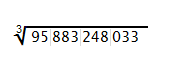Step 1: Starting at the left, take the cube root of the first group of numbers (may be a one-, two-, or three-digit number) and round down to the nearest whole digit if you get a decimal. In this example, the first group of numbers is 95 and its cube root is about 4.56, which you round down to 4. Write this digit above the first group of digits, above the radical bar. Cube the digit, write that number below the first group of digits, and subtract. Bring down the next triple of digits.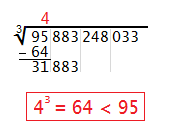Step 2: Call the new number at the bottom C and the digit above the radical bar P. You want to find the largest digit N such that (300P²+30PN+N²)N ≤ C. To find a candidate for N, compute C/(300P²) and round down to the nearest whole digit. Test your candidate to make sure it is the largest digit that satisfies (300P²+30PN+N²)N ≤ C; you may have to adjust it up or down. Once you find N, write it above the second triple of digits, above the radical bar. Then compute (300P²+30PN+N²)N, write it below C, subtract, and bring down the next triple of digits.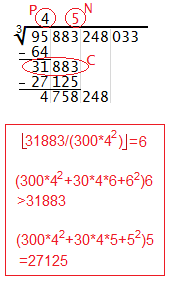Step 3: Repeat the process outlined in Step 2, except this time your value of C is the new number at the bottom (4758248 in this example), and your value of P is the new number above the radical bar (45 in this example). For instance, if C = 4758248 and P = 45, you first compute 4758248/(300*45²), which equals 7 when rounded down. Then you check whether (300*45²+30*45*7+7²)(7) is less than or equal to 4758248. It turns out the answer is yes, so you write 7 above the radical bar. And since (300*45²+30*45*7+7²)(7) = 4318993, you compute the difference 4758248 - 4318993, which equals 439255. When you drop down the next three digits, you get 439255033.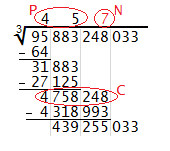Step 4: If your original number turns out to be a perfect cube, the process will terminate when you get a difference of 0 at the end. In this example, it turns out that 4577³ = 95883248033 exactly. If your number is not a perfect cube and you want to compute its cube root to the nearest tenths or hundredths, you must append several zeros to the right of the final digit, in sets of three, and continue the process outlined above.